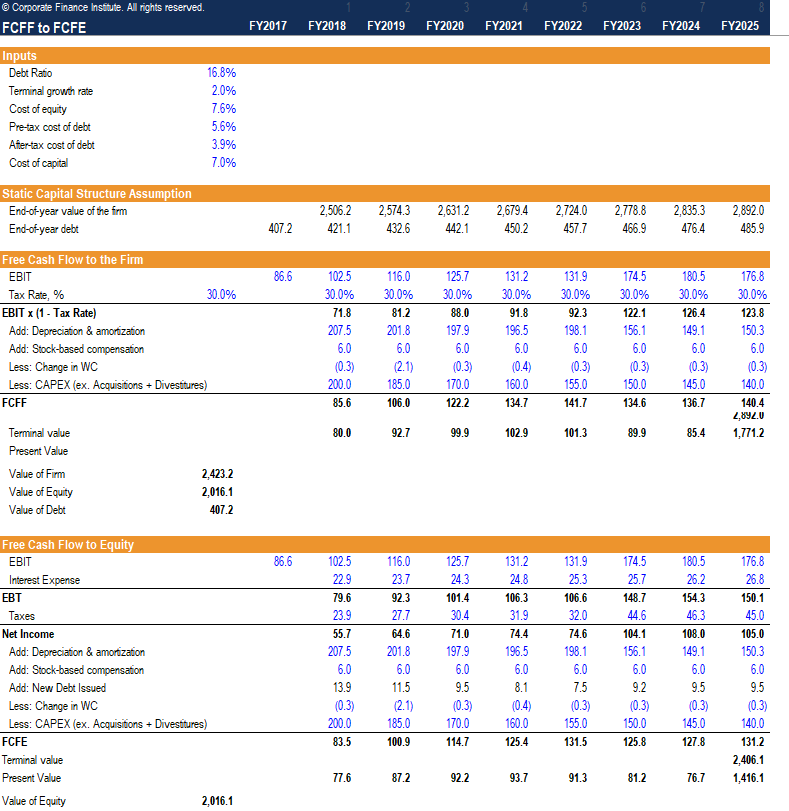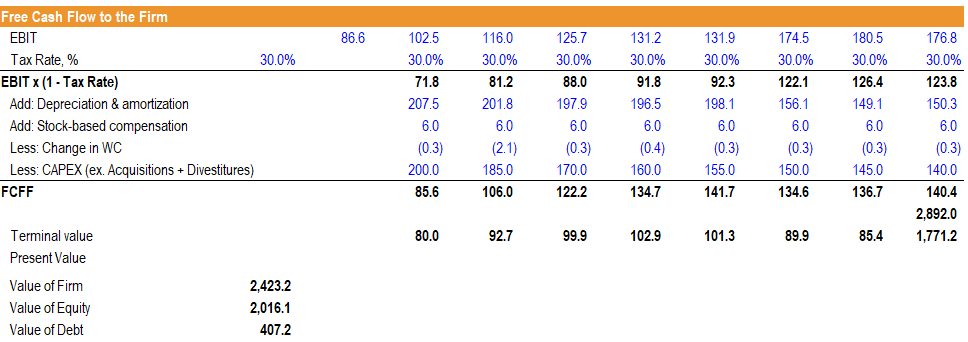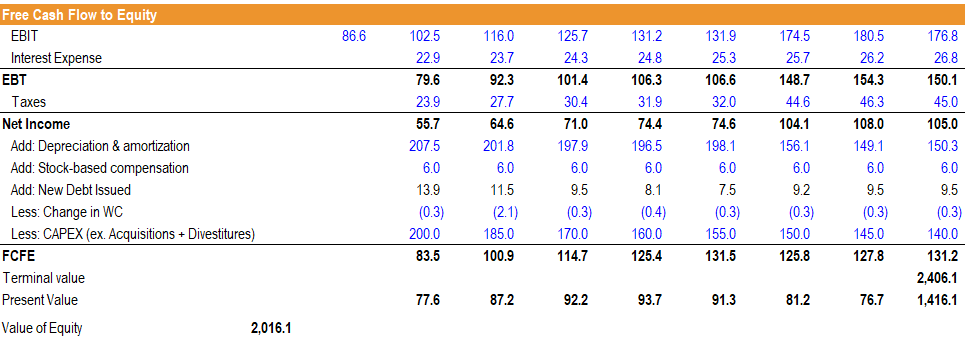# FCFF vs FCFE Reconciliation

Guide to reconciling FCFF to FCFE

## FCFF vs FCFE Reconciliation Template

Free Cash Flow to the Firm (FCFF) is the cash flow available to all the firm’s suppliers of capital once the firm pays all operating and investing expenditures needed to sustain the firm’s existence. Operating expenditures include the fixed and variable costs, inputs required by a company to generate its primary economic benefit, and revenues. Investing activities include expenditures by a company in its property, plant & equipment, as well as intangible assets, on top of short-term working capital investments such as inventory, and the deferred payments and receipts of revenue in its accounts payable and receivable. The remaining cash flows are those that are available to the firm’s suppliers of capital, namely its stockholders and bondholders. This guide includes a template to reconcile FCFF vs FCFE.Free Cash Flow to Equity (FCFE) is the cash flow available to the firm’s stockholders. The cash flows are inclusive of all of the expenses above, as well as the net cash outflows to its bondholders. Using the dividend discount model is similar to the FCFE approach, as both forms of cash flows represent the cash flows available to stockholders. In general, the FCFE method is preferred when the dividend policy of the firm is not stable, or when an investor owns a controlling interest in the firm.

All three types of cash flows can be used to determine the intrinsic value of equity, and ultimately, the firm’s intrinsic stock price. The primary difference in valuation methods lies in how the cash flows are discounted, and the primary insight to be gained is that all three methods account for the inclusion of debt in a firm’s capital structure, albeit in different ways. Utilizing the provided worksheet, we can illustrate how these cash flows reconcile, how they are valued, and when each type is appropriate for valuation.

### Reconciling FCFF vs FCFE

To reconcile FCFF to FCFE, we must first make a set of important assumptions about the firm’s financials and its capital structure. We must assume that the capital structure of the firm will not change over time. It is an important assumption because if the firm’s capital structure changes, the marginal cost of capital will also change. Second, we must work with the same fundamental financial variables across both methods and apply the same tax rates and reinvestment requirements across both methods.

### FCFF vs FCFE Reconciliation Template

Follow the steps below to complete the template of FCFF vs FCFE:

##### Step 1

Enter the base inputs of the calculation worksheet. These include the firm’s debt ratio (which is assumed to remain static), the pre-tax cost of debt, the tax rate, the cost of equity and the terminal growth rate.

• The free cash flow to the firm is determined in each year by converting the company’s operating profit (EBIT) to NOPAT by multiplying by (1 – tax rate) adding back non-cash expenses and subtracting net firm reinvestment (working capital and capital expenditures).
• The present value of the firm’s FCFF and terminal value is added together to find the intrinsic value of the firm as of today. Assuming the company has zero cash, subtracting the value of debt from the firm’s valuation will yield the value of equity.##### Step 2

The static capital structure assumption section calculates the value of the firm in each respective forward year, using each forward year as the present year to calculate different present values. Then, the end-of-year debt assumption is computed by taking the product of each forward year’s firm value and the static debt to capital ratio. From this point we can begin computing the firm’s equity value standalone.

• We begin at the firm’s operating profit (EBIT) and subtract the firm’s interest expense. The interest expense is calculated by taking the product of the firm’s cost of debt and its year-end debt in each forward year. The difference yields the firm’s earnings before tax (EBT).
• The tax expense is calculated by taking the product of the tax rate used in the FCFF section and the earnings before tax in each forward year. The difference yields the firm’s net income.
• The free cash flow to equity is computed by taking the firm’s net income in each forward year, adding back non-cash charges, subtracting net firm reinvestment just as in FCFF with one key difference. We must also add back the net increase in debt, as this is new capital that is available for the firm.
• The present value of the firm’s FCFE and terminal value of its equity is added together to find the intrinsic value of the firm as of today.### Summary

The first thing we notice is that we arrive at the same equity valuation in both methods. The first difference in these two methods is the discount rate applied. The FCFF method utilizes the weighted average cost of capital (WACC), whereas the FCFE method utilizes the cost of equity only.

The second difference in these two methods is the treatment of debt. The FCFF method subtracts debt at the very end to arrive at the intrinsic value of equity, whereas the FCFE method integrates interest payments and net additions to debt to arrive at FCFE.

### More Resources

Thank you for reading this guide to reconciling FCFF vs FCFE. CFI is the official provider of the Financial Modeling & Valuation Analyst (FMVA) certification program for financial analysts. To continue advancing your career, these additional resources will be helpful:

• Weighted Average Cost of Capital (WACC)
• Cost of Equity
• Capital Structure
• Equity Value

### Financial Analyst Certification

Become a certified Financial Modeling and Valuation Analyst (FMVA)® by completing CFI’s online financial modeling classes and training program!